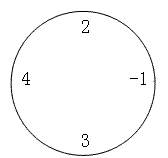P1043 数字游戏

• 3.7K通过
• 8.6K提交
• 题目提供者 CCF_NOI
• 评测方式 云端评测
• 标签 前缀和 动态规划,动规,dp 搜索 NOIp普及组 2003
• 难度 普及+/提高
• 时空限制 1000ms / 128MB
• 提示：收藏到任务计划后，可在首页查看。

题目描述

丁丁最近沉迷于一个数字游戏之中。这个游戏看似简单，但丁丁在研究了许多天之后却发觉原来在简单的规则下想要赢得这个游戏并不那么容易。游戏是这样的，在你面前有一圈整数（一共$n$个），你要按顺序将其分为$m$个部分，各部分内的数字相加，相加所得的$m$个结果对$10$取模后再相乘，最终得到一个数$k$。游戏的要求是使你所得的k最大或者最小。

例如，对于下面这圈数字（$n=4,m=2$）：要求最小值时，$((2-1) \bmod 10)×((4+3) \bmod 10)=1×7=7$，要求最大值时，为$((2+4+3) \bmod 10)×(-1 \bmod 10)=9×9=81$。特别值得注意的是，无论是负数还是正数，对$10$取模的结果均为非负值。

丁丁请你编写程序帮他赢得这个游戏。

输入输出格式

输入格式：

输入文件第一行有两个整数，$n(1≤n≤50)$和$m(1≤m≤9)$。以下$n$行每行有个整数，其绝对值$\le 10^4$，按顺序给出圈中的数字，首尾相接。

输出格式：

输出文件有$2$行，各包含$1$个非负整数。第$1$行是你程序得到的最小值，第$2$行是最大值。

输入输出样例

输入样例#1： 复制
4 2
4
3
-1
2

输出样例#1： 复制
7
81

提示
标程仅供做题后或实在无思路时参考。
请自觉、自律地使用该功能并请对自己的学习负责。
如果发现恶意抄袭标程，将按照I类违反进行处理。